# Diffraction, mathematical theory of

A branch of mathematical physics dealing with the problems involved in the mathematical description of wave phenomena. This definition also includes geometrical optics, but this discipline is traditionally considered as an independent branch of mathematical physics. The fundamental partial differential equations which describe wave processes are the Maxwell equations, the equations of dynamic problems of elasticity theory, the wave equation (in two spatial variables it describes vibrations of membranes; in three spatial variables it represents the propagation of sound), the equations of hydrodynamics and several others.

## Problem formulation in the mathematical theory of diffraction.

These problems may be discussed taking the wave equation as an example; problems for other equations describing wave processes are formulated in a similar manner.

Transient problems of diffraction are, essentially, mixed problems (cf. Mixed problem), i.e. problems involving initial and boundary conditions for the wave equation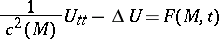(1)

in the domain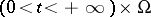, whereis a domain in the plane or in three-dimensional space; also(2)

on the boundaryof the domain, in the simplest cases,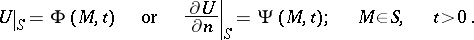(3)

Here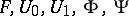are given functions. The coefficient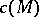on certain surfaces (in the three-dimensional case) or lines (in the planar case) may have jumps; in such a case it is necessary to specify, in addition, "conjugate conditions" for, which interconnect the values of the functionand its derivatives on different sides of the surface across whichhas a jump. That such a formulation of problems of this kind is correct is proved by a priori estimates of the unknown solution in different norms, using the concept of compactness. The detailed problems of the description of the singularities of the solutions, the behaviour of the solutions as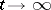, and the development of algorithms useful in practice for a numerical solution are still far from being finally solved.

Stationary problems of diffraction. An important role in the mathematical theory of diffraction is played by solutions that depend harmonically on time, i.e. solutions of equation (1) of the form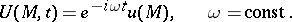(4)

The parametercorresponds to the circular frequency. The functions,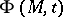and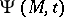(see formulas (1) and (3)) should also show a harmonic dependence on time: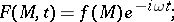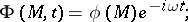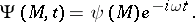Equation (1) and the boundary conditions (3) give(5)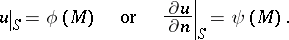(6)

Of major importance for bounded domainsis the problem of finding non-trivial solutions of equation (5) for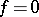in the case of homogeneous boundary conditionsor. This is the so-called problem of finding the eigen functions. However, it is the solution of equation (5) under the boundary conditions (6) for unbounded domainswhich is of fundamental interest in the mathematical theory of diffraction. The conditions (5) and (6) alone are insufficient for the determination of a function, and "conditions at infinity" must also be specified.

The most frequent physical formulation of the problem of diffraction is the following: It is assumed that the solutionof equation (5) is given as the sumof two functions, whereis a known function (the "incident wave" ), while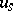is the "scattered" or the diffraction-generated wave. The wavemust not contain waves issuing from infinity; this physical condition leads to the conditions at infinity just mentioned. The corresponding conditions in the situation whereis the exterior of a bounded domain,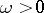,is constant outside a sphere of a sufficiently large radius, while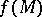is a function of compact support, are known as the radiation conditions. If the radiation conditions are met, the problem (5), (6) is well-posed; this is proved either by reducing the problems to integral equations or by a priori estimates. Other methods for finding the unique solution of problem (5), (6) are based on the limiting-amplitude principle and on the limit-absorption principle. Ifis the exterior of a bounded domain, the two principles yield the same solution as the radiation conditions. No general theory of problems involving the Helmholtz equation in the case of unbounded domains has been developed. The limit-absorption principle will yield solutions of physical interest in all cases of practical importance.

If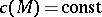, solutions of the Dirichlet problem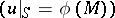and the Neumann problem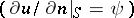may be obtained in the form of double- and single-layer potentials, respectively: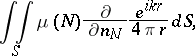For the unknown density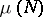a Fredholm integral equation of the second kind is obtained. In proving the solvability of the Dirichlet and the Neumann problem the difficulties encountered are completely similar to the classical difficulties involved in solving the exterior Dirichlet problem for the Laplace equation by methods of potential theory.

## Methods for solving problems in the mathematical theory of diffraction.

Of major importance in problems of the mathematical theory of diffraction is the ratio between the wavelength and the characteristic dimensions of the body in the vicinity of which the wave process is studied. If the wavelength is large (small) as compared with the characteristic dimensions of the body, one speaks of the diffraction of long (short) waves. In the former cases the quantityin equation (5) may be regarded as a small parameter, which makes it possible to apply in this case the different variants of perturbation theory. The solution of the Laplace equation may be taken as the first approximation. Ordinary numerical methods of mathematical physics — e.g. variational methods and numerical solution of the integral equations of potential theory — are also applicable in this case. For domains of special type the problems in the mathematical theory of diffraction may be solved in an explicit form, i.e. the solutions are given in the form of series or integrals containing special functions. The most important tools for this purpose are the method of separation of variables, or the Fourier method, and the Wiener–Hopf method.

Obtaining numerical results for explicit solutions of problems in the mathematical theory of diffraction was formerly successful in cases of long waves only (the shorter the wave, the poorer the convergence of the series and the integrals). As the computing techniques developed, many problems were solved also in domains where the wavelength is not linearly proportional to the characteristic dimension. The most effective methods for such a purpose consist in reducing the problems of diffraction to integral and integro-differential equations, and the different variants of projection methods.

## Asymptotic methods in the mathematical theory of diffraction.

If the wavelength is small, numerical solution of the problems in diffraction is very difficult, even when computers are used. The most important methods in this category are asymptotic ones, which have the additional advantage of permitting certain general conclusions to be made about the problem under investigation. Asymptotic methods are certain methods yielding the approximate value of the unknown functions. They are based on physical ideas and formal transformations, mostly without a rigorous foundation. One of the first methods for finding approximate solutions of short-wave problems in the form of some quadrature was the Kirchhoff method, which is widely used to this very day in solving practical problems. Just as important is the ray method, which gives rise to a number of methods for finding the asymptotics of many problems of diffraction. As a rule, the constructions involved in the ray method are easily performed, provided the relevant ray field is regular. If, on the other hand, the field has any singularities, the resulting situation is typical of the boundary-layer theory: The asymptotics of solutions are known everywhere except for very small neighbourhoods of the points at which the ray field is no longer regular. It is only necessary to find it in this small domain, i.e. in the boundary layer. The corresponding analogue of the boundary-layer method is known as the parabolic-equation method, which is often employed both in the numerical solution of problems in the mathematical theory of diffraction and in deriving asymptotic formulas.

Important asymptotic formulas for solving problems in the mathematical theory of diffraction may also be obtained when an explicit expression of the solution is known. Using a well-developed method (the so-called Watson transformation), this explicit solution is converted to a contour integral, the asymptotics of which is sought with the aid of either the saddle point method or the residue theorem. It is interesting to note that the asymptotic expressions thus obtained may be used as guides, "models" , to "guess" the form of asymptotic expansions in problems of diffraction the explicit solution of which is unknown. Such constructions are characteristic of the "method of canonical problemsmethod of canonical problems" , which is a development of the parabolic-equation method.